Case Based Questions (MCQ)

Chapter 1 Class 10 Real Numbers
Serial order wise

## Question A seminar is being conducted by an Educational Organization, where the participants will be educators of different subjects. The number of participants in Hindi, English and Mathematics are 60, 84 and 108 respectively.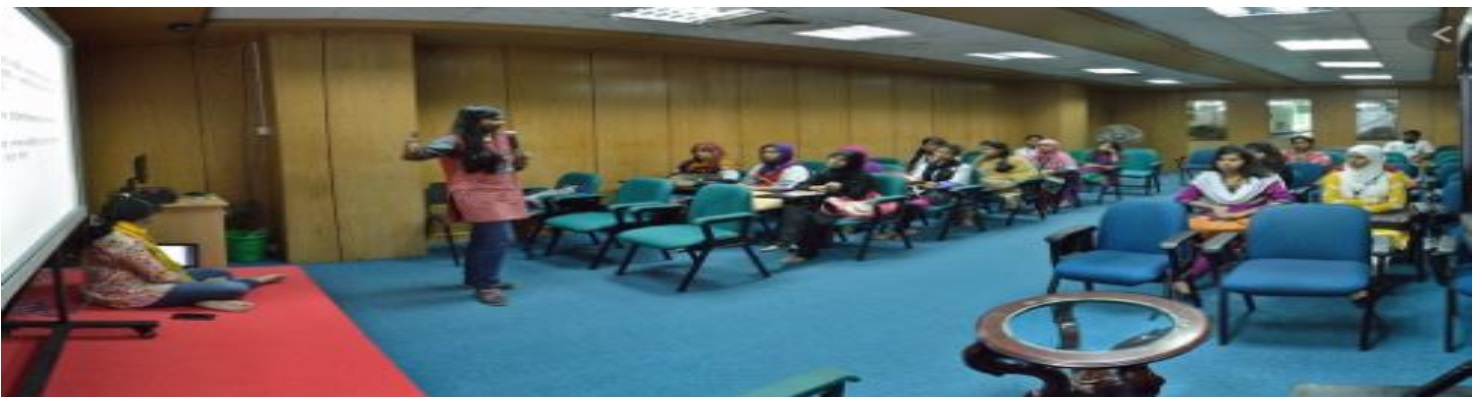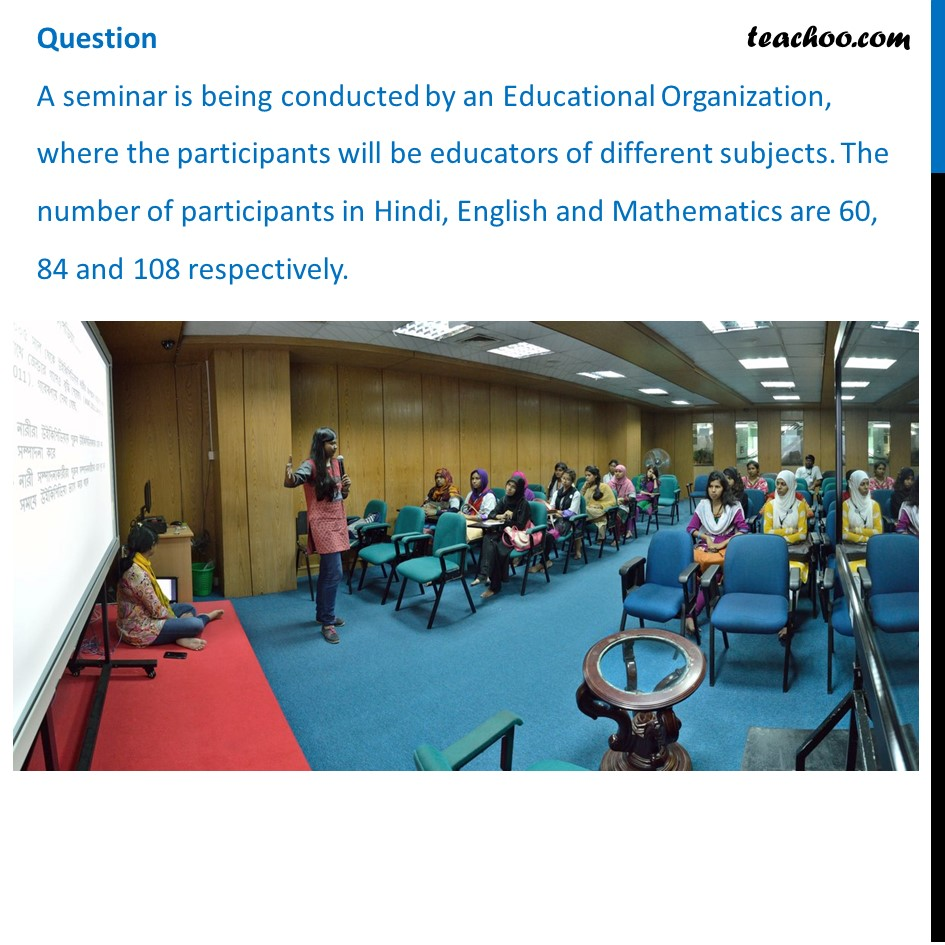## Question 1 In each room the same number of participants are to be seated and all of them being in the same subject, hence maximum number participants that can accommodated in each room are (a) 14   (b) 12   (c) 16  (d) 18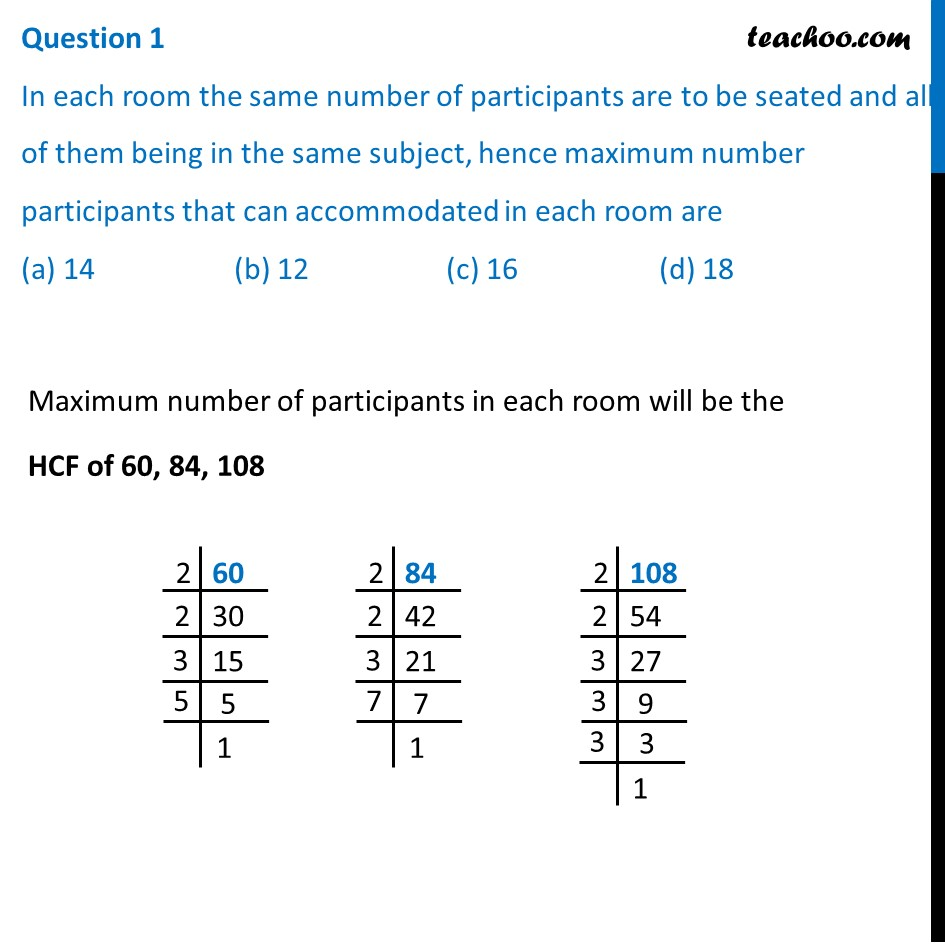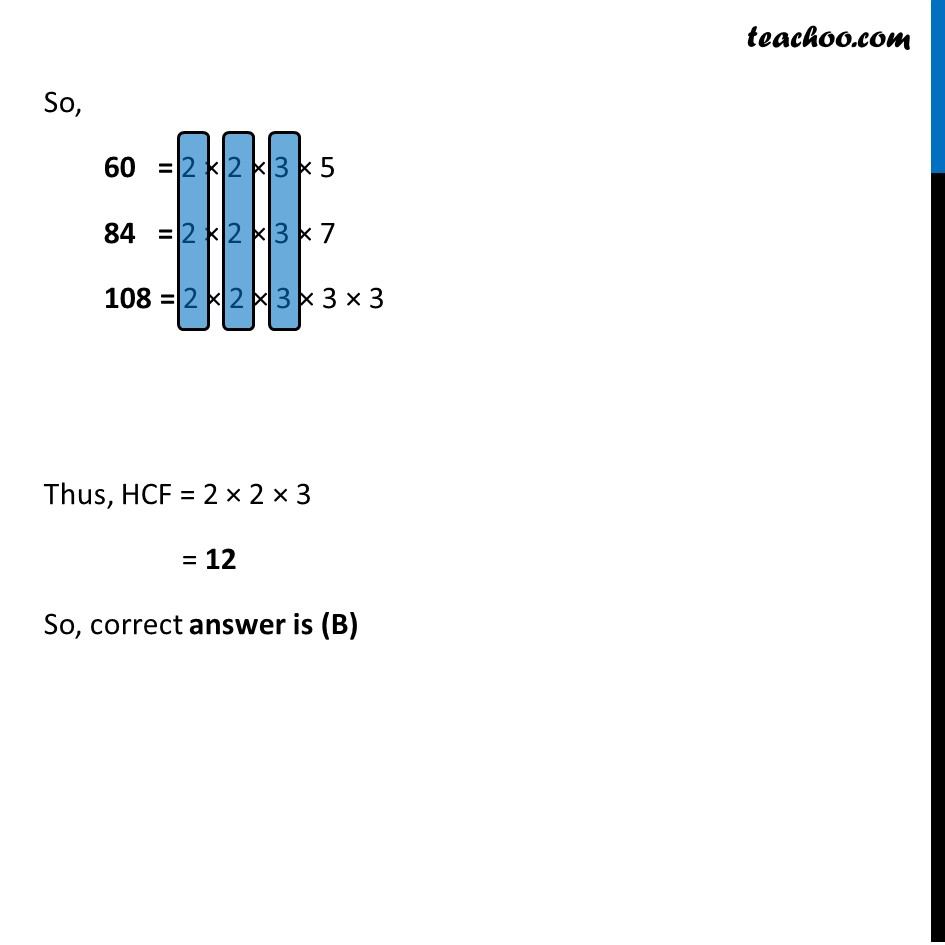## Question 2 What is the minimum number of rooms required during the event? (a) 11  (b) 31   (c) 41  (d) 21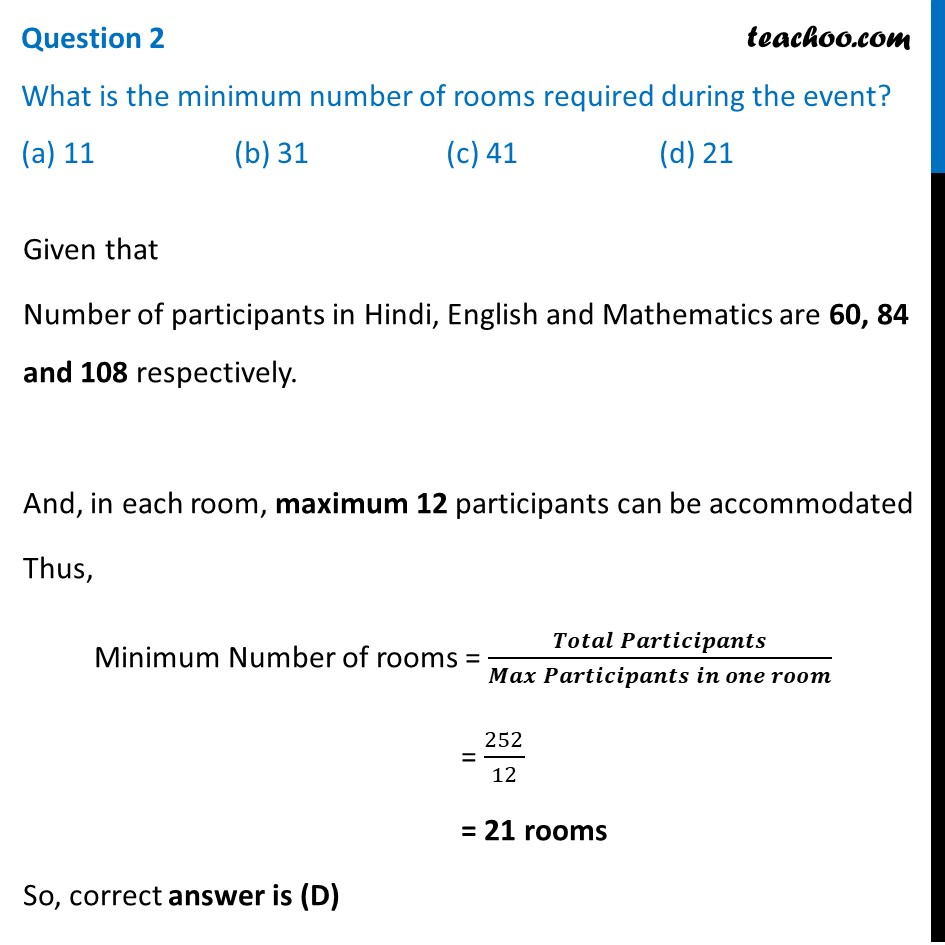## Question 3 The LCM of 60, 84 and 108 is (a) 3780  (b) 3680  (c) 4780   (d) 4680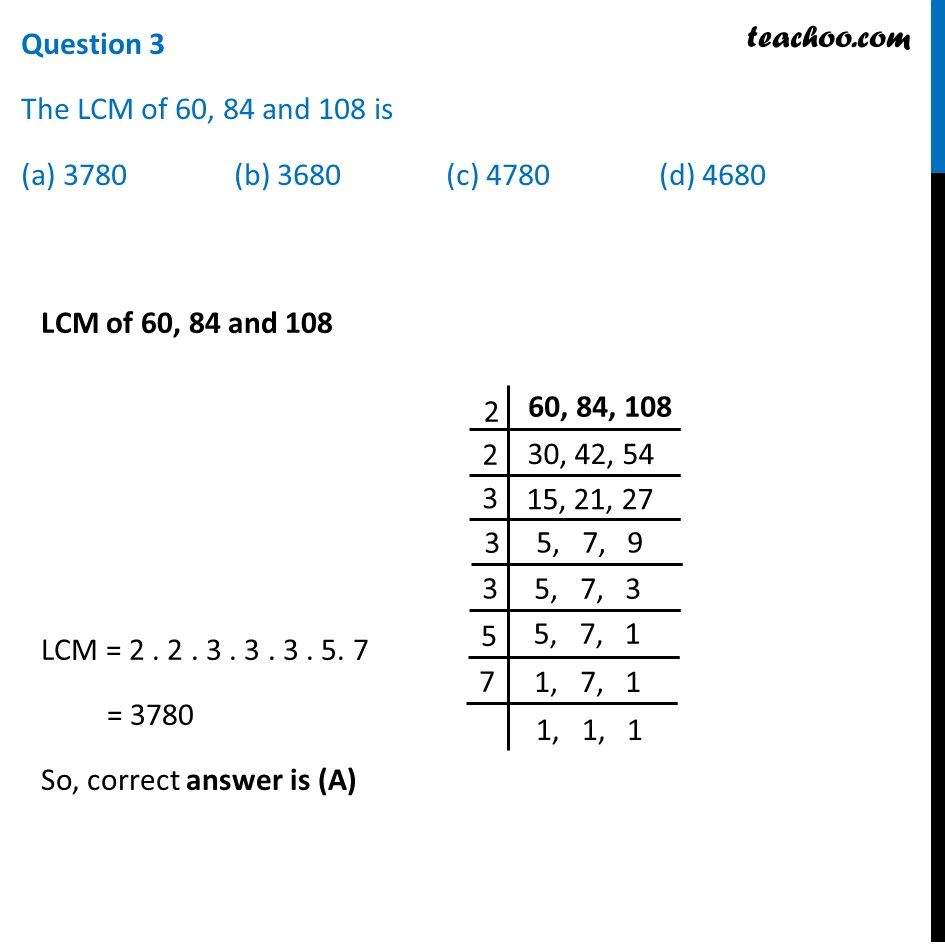## Question 4 The product of HCF and LCM of 60, 84 and 108 is (a) 55360  (b) 35360  (c) 45500  (d) 45360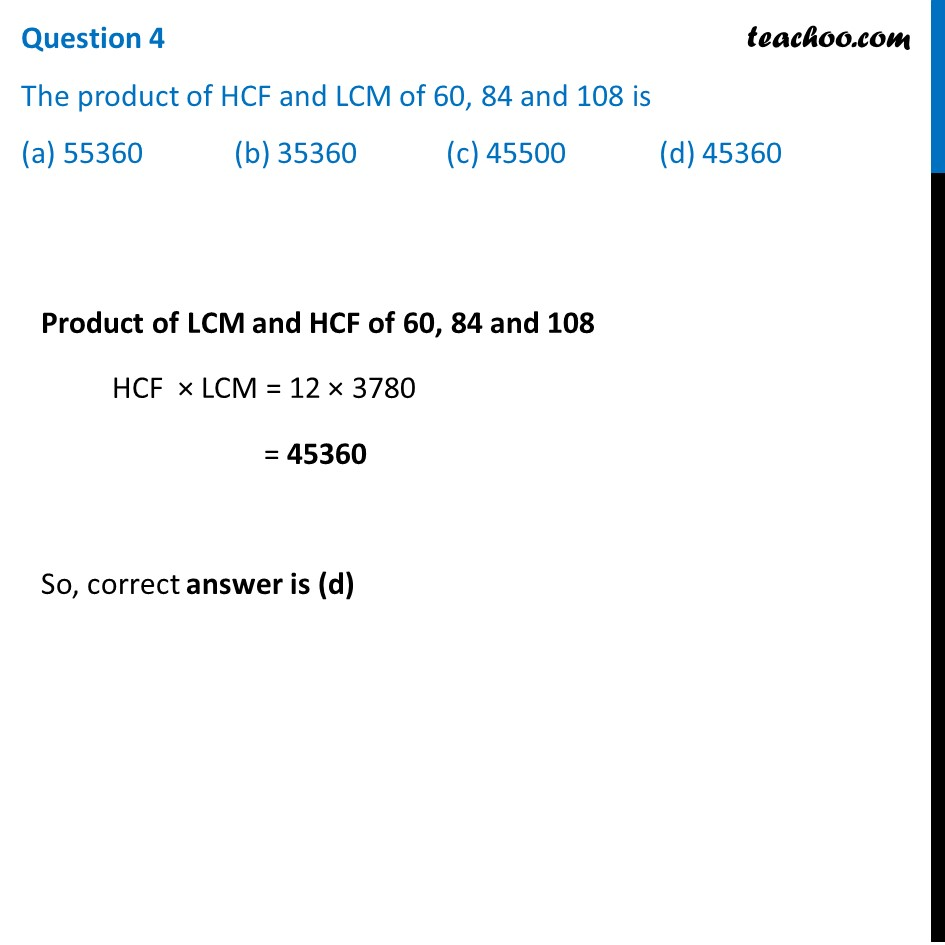## Question 5 108 can be expressed as a product of its primes as (a) 2 3   × 3 2 (b) 2 3 × 3 3 (c) 2 2 × 3 2 (d) 2 2 × 3 3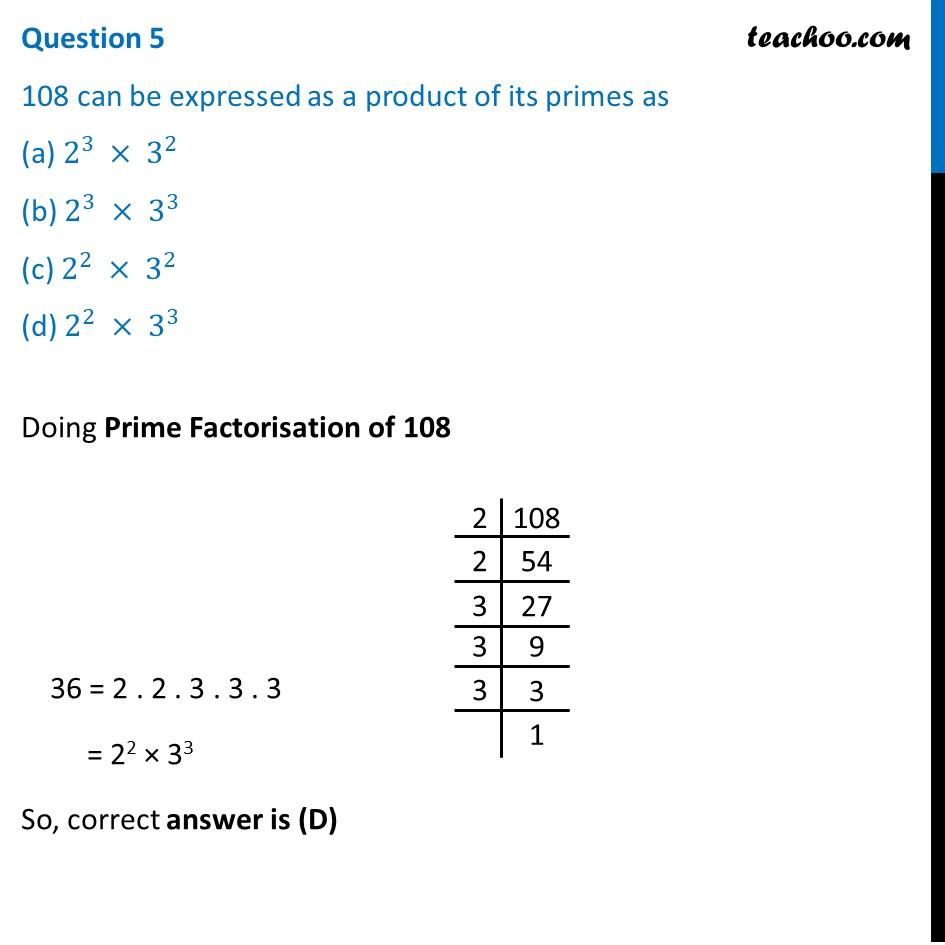Learn in your speed, with individual attention - Teachoo Maths 1-on-1 Class

### Transcript

Question A seminar is being conducted by an Educational Organization, where the participants will be educators of different subjects. The number of participants in Hindi, English and Mathematics are 60, 84 and 108 respectively. Question 1 In each room the same number of participants are to be seated and all of them being in the same subject, hence maximum number participants that can accommodated in each room are (a) 14 (b) 12 (c) 16 (d) 18 Maximum number of participants in each room will be the HCF of 60, 84, 108 So, 60 = 2 × 2 × 3 × 5 84 = 2 × 2 × 3 × 7 108 = 2 × 2 × 3 × 3 × 3 Thus, HCF = 2 × 2 × 3 = 12 So, correct answer is (B) Question 2 What is the minimum number of rooms required during the event? (a) 11 (b) 31 (c) 41 (d) 21 Given that Number of participants in Hindi, English and Mathematics are 60, 84 and 108 respectively. And, in each room, maximum 12 participants can be accommodated Thus, Minimum Number of rooms = (𝑻𝒐𝒕𝒂𝒍 𝑷𝒂𝒓𝒕𝒊𝒄𝒊𝒑𝒂𝒏𝒕𝒔)/(𝑴𝒂𝒙 𝑷𝒂𝒓𝒕𝒊𝒄𝒊𝒑𝒂𝒏𝒕𝒔 𝒊𝒏 𝒐𝒏𝒆 𝒓𝒐𝒐𝒎) = 252/12 = 21 rooms So, correct answer is (D) Question 3 The LCM of 60, 84 and 108 is (a) 3780 (b) 3680 (c) 4780 (d) 4680LCM of 60, 84 and 108 LCM = 2 . 2 . 3 . 3 . 3 . 5. 7 = 3780 So, correct answer is (A) Question 4 The product of HCF and LCM of 60, 84 and 108 is (a) 55360 (b) 35360 (c) 45500 (d) 45360 Product of LCM and HCF of 60, 84 and 108 HCF × LCM = 12 × 3780 = 45360 So, correct answer is (d) Question 5 108 can be expressed as a product of its primes as (a) 2^3 × 3^2 (b) 2^3 × 3^3 (c) 2^2 × 3^2 (d) 2^2 × 3^3 Doing Prime Factorisation of 108 36 = 2 . 2 . 3 . 3 . 3 = 22 × 33 So, correct answer is (D)